# 1 Introduction

创新：基于采样和聚合的算法。

## 1.1 Transductive Learning

即直推式学习，只能使用已经观测到的数据（有标签），对于没有标签的节点在训练过程中只能忽略（不适用结构信息和属性信息）。

缺点：一旦有新的节点出现，直推式学习需要重新训练模型。

## 1.2 Inductive Learning

即归纳式学习，已经预先观察了所有数据，含训练和测试数据集。 从已经观察到的数据集中学习，然后预测测试数据集的标签。 即过程会利用这些不知道数据标签的测试集数据的模式和其他信息。

主要观点是：节点的嵌入可以通过一个共同的聚合邻居节点信息的函数得到，在训练时只要得到这个聚合函数，就可以将其泛化到未知的节点上。

# 2 GraphSAGE Method

GraphSAGE 的核心思想：不是试图学习一个图上所有 Node Embedding，而是学习一个为每个 Node 产生 Embedding 的映射（即产生一个通用的映射函数）。

本文提出的 GraphSAGE（Inductive Method） 可以利用所有图中存在的结构特征（如：节点度，邻居信息），去推测 Unseen Node 的节点 Embeeding。

举例如下：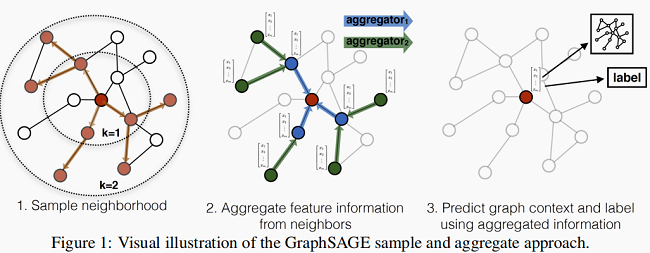1. 先对邻居随机采样，降低计算复杂度（Figure 1 ：一跳邻居采样数=3，二跳邻居采样数=5）
2. 生成目标节点 Emebedding：先聚合2跳邻居特征，生成一跳邻居 Embedding，再聚合一跳邻居 Embedding，生成目标节点 Embedding，从而获得二跳邻居信息。
3. 将 Embedding 作为全连接层的输入，预测目标节点的标签。

## 2.1 Embedding generation algorithm

GraphSAGE 算法如下：注意：$K$ 控制着跳数，本文这边取 $K=2$。

举例：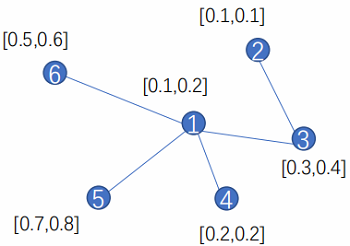这里以节点 $1$ 为例，采用均值聚合。

对于节点  $1$ ，它相连的邻居为 ${3,4,5,6}$。（这里以聚合所有邻居信息为例）

对于算法中的第 4 步：$h_{\mathcal{N}(1)}^{1} \leftarrow A G G R E G A T E\left(\left\{h_{3}^{0}, h_{4}^{0}, h_{5}^{0}, h_{6}^{0}\right\}\right)$：

$h_{\mathcal{N}(1)}^{1}=A G G R E G A T E\left(\left\{h_{3}^{0}, h_{4}^{0}, h_{5}^{0}, h_{6}^{0}\right\}\right)=\operatorname{Mean}([0.3,0.4],[0.2,0.2],[0.7,0.8],[0.5,0.6]$

对于算法中的第 5 步：$h_{1}^{1} \leftarrow \sigma\left(W^{1} \cdot \operatorname{CONCAT}\left(h_{1}^{0}, h_{\mathcal{N}(1)}^{1}\right)\right)$ ：

$\left.h_{1}^{1}=W \cdot \operatorname{CONCAT}\left(h_{1}^{0}, h_{\mathcal{N}(1)}^{1}\right)\right)=W \cdot[0.1,0.2,0.425,0.5]$

改进：聚合部分邻居

• 对于节点 $1$，比如我们要聚合其 $3$ 个邻居的信息，那就按均匀分布随机在其邻居集合中选择 $3$ 个邻居节点。（节点不重复）
• 对于节点 $1$，比如我们要聚合其 $6$ 个邻居的信息，那就先聚合其所有邻居一次（$5$ 个邻居），然后在按均匀分布随机在其邻居集合中选择 $1$ 个邻居节点。（节点重复）

注意点：上述提到 $K$ 控制着跳数。

举例：【$K=2,S_1 =2,S_2 = 3$】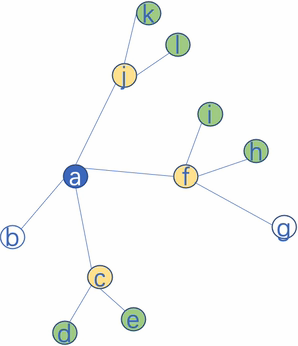本文实验说明聚合邻居数最好满足： $S_{1} \cdot S_{2} \leq 500$。

基于 minibatch 版本的 GraphSAGE 算法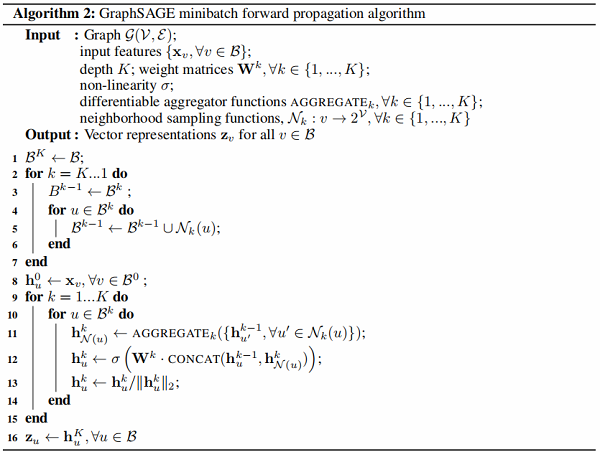举例：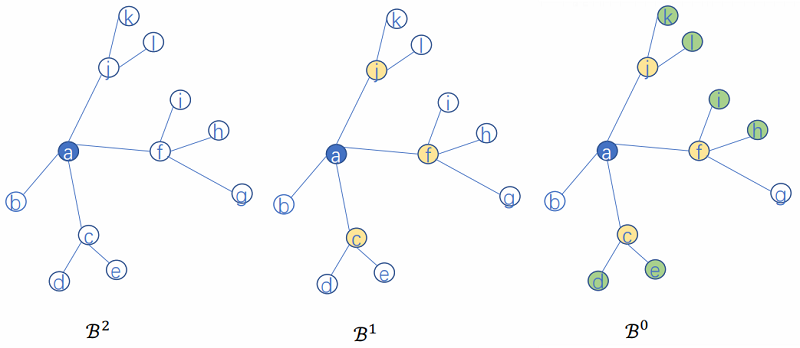考虑：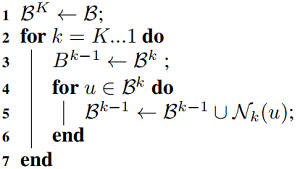假设：$K=2, S_1=2, S_2=3$，$\mathcal{B}^{2}=\{a\}$

那么：

$\mathcal{B}^{1}=\{a\} \cup \mathcal{N}_{2}(a)=\{a\} \cup\{c, f, j\}$

$\mathcal{B}^{0}=\{a\} \cup\{c, f, j\} \cup \mathcal{N}_{1}(\{c, f, j\})=\{a\} \cup\{c, f, j\} \cup\{d, e, i, h, k, l\}$

考虑：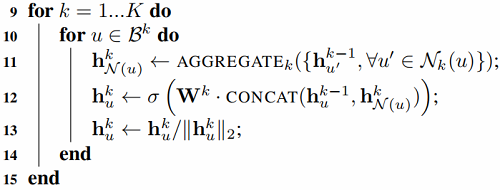$\begin{array}{l}\mathcal{B}^{1}=\{a\} \cup \mathcal{N}_{2}(a)=\{a\} \cup\{c, f, j\} \\\mathcal{N}_{1}(c)=\{d, e\} \\h_{\mathcal{N}(c)}^{1} \leftarrow A G G R E G A T E_{1}\left\{h_{d}^{0}, h_{e}^{0}\right\} \\h_{c}^{1} \leftarrow \sigma\left(W^{1} \cdot \operatorname{CONCAT}\left(h_{c}^{0}, h_{\mathcal{N}(1)}^{1}\right)\right)\end{array}$

## 2.2 Learning the parameters of GraphSAGE

损失函数分为基于图的无监督损失有监督损失

• 基于图的无监督损失：目标是使节点 $u$ 与 “邻居” $v$ 的 Embedding 相似，与无边相连的节点 $v_n$ 不相似。

$J_{\mathcal{G}}\left(\mathbf{z}_{u}\right)=-\log \left(\sigma\left(\mathbf{z}_{u}^{\top} \mathbf{z}_{v}\right)\right)-Q \cdot \mathbb{E}_{v_{n} \sim P_{n}(v)} \log \left(\sigma\left(-\mathbf{z}_{u}^{\top} \mathbf{z}_{v_{n}}\right)\right)$

其中：

• 节点 $v$  是节点 $u$  经过固定长度的 Random walk 到达的邻居节点；
• $v_{n} \sim P_{n}(u)$  表示负采样：节点 $v_{n}$  是从节点 $u$  的负采样分布 $P_{n}$  采样的， $Q$  为采样样本数；
• 基于图的有监督损失：无监督损失函数的设定来学习节点 Embedding 可以供下游多个任务使用，若仅使用在特定某个任务上，则可以替代上述损失函数符合特定任务目标，如交叉熵。

## 2.3 Aggregator Architectures

由于节点是无序的，所以聚合器需要满足排列不变性。

排列不变性（permutation invariance）：指输入的顺序改变不会影响输出的值。

• Mean aggregator

$h_{v}^{k}=\sigma\left(W^{k} \cdot \operatorname{mean}\left(\left\{h_{v}^{k-1}\right\} \cup\left\{h_{u}^{k-1}, \forall u \in N(v)\right\}\right)\right.$

也可以是：
$\begin{array}{c}h_{N(v)}^{k}=\operatorname{mean}\left(\left\{h_{u}^{k-1}, u \in N(v)\right\}\right) \\h_{v}^{k}=\sigma\left(W^{k} \cdot C O N C A T\left(h_{v}^{k-1}, h_{N(u)}^{k}\right)\right)\end{array}$
• LSTM aggregator

LSTM函数不符合 "排列不变性" 的性质，需要先对邻居随机排序，然后将随机的邻居序列 Embedding $\left\{x_{t}, t \in N(v)\right\}$  作为 LSTM 输入。

• Pooling aggregator

一个 element-wise max pooling 操作应用在邻居集合上来聚合信息：
$\text { AGGREGATE }_{k}^{\mathrm{pool}}=\max \left(\left\{\sigma\left(\mathbf{W}_{\text {pool }} \mathbf{h}_{u_{i}}^{k}+\mathbf{b}\right), \forall u_{i} \in \mathcal{N}(v)\right\}\right)$

$\mathbf{h}_{v}^{k} \leftarrow \sigma\left(\mathbf{W}^{k} \cdot \operatorname{CONCAT}\left(\mathbf{h}_{v}^{k-1}, \mathbf{h}_{\mathcal{N}(v)}^{k}\right)\right)$

# 3 Experiments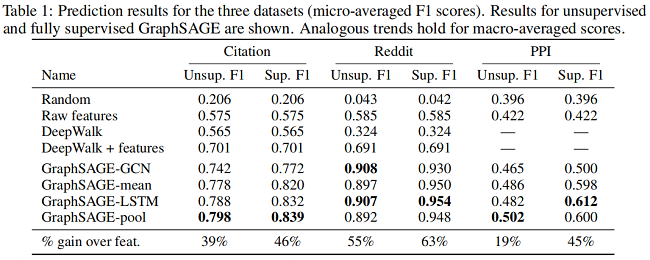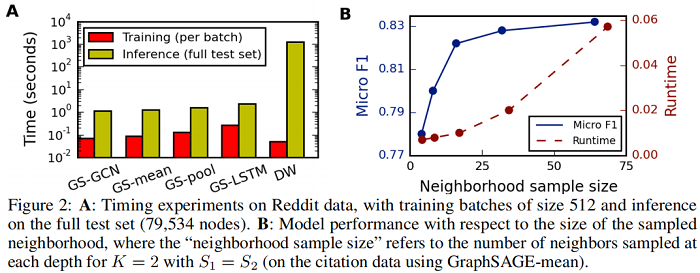『总结不易，加个关注呗！』

posted @ 2022-01-17 08:24  Learner-  阅读(148)  评论(0编辑  收藏  举报